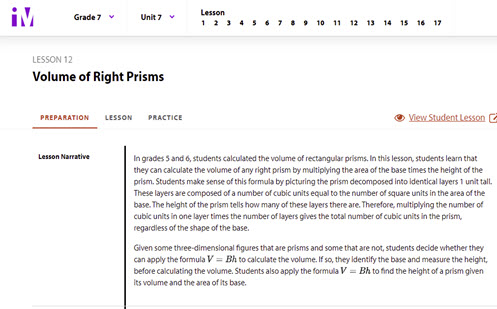Go to website

# Volume of right prisms

In this lesson, students learn to calculate the volume of any right prism by multiplying the area of the base times the height of the prism. They derive this formula by picturing the prism decomposed into identical layers 1 unit tall.

Year level(s) Year 8
Audience Teacher
Format Web page
Teaching strategies and pedagogical approaches Explicit teaching
Keywords Area, Cylinder, Box, Solid, Net, Volume, Surface area

## Curriculum alignment

Curriculum connections Critical and creative thinking, Numeracy
Strand and focus Space
Topics Area, volume and surface area
AC: Mathematics (V9.0) content descriptions
AC9M8M06

Use Pythagoras' theorem to solve problems involving the side lengths of right-angled triangles

Numeracy progression Understanding geometric properties (P7)
Understanding units of measurement (P10)
Multiplicative strategies (P9)